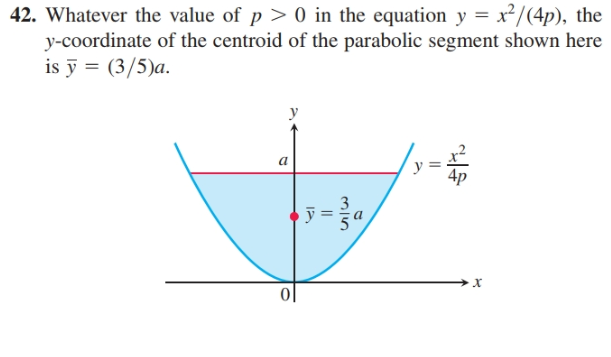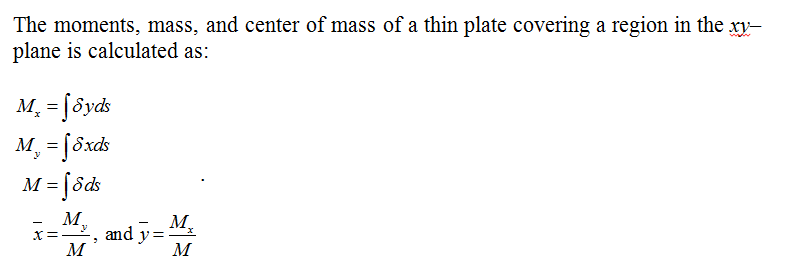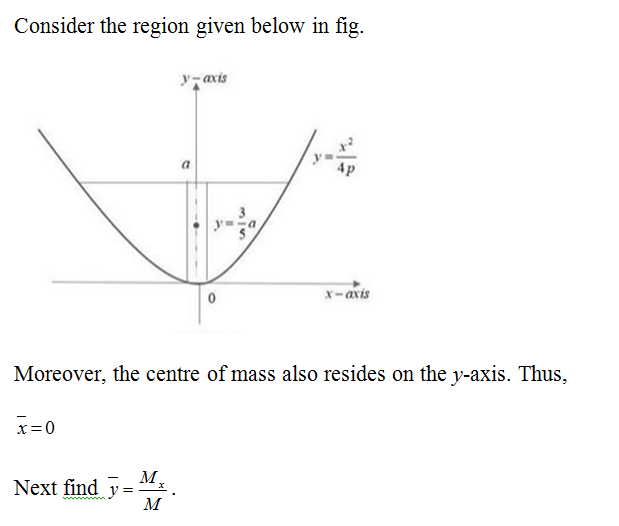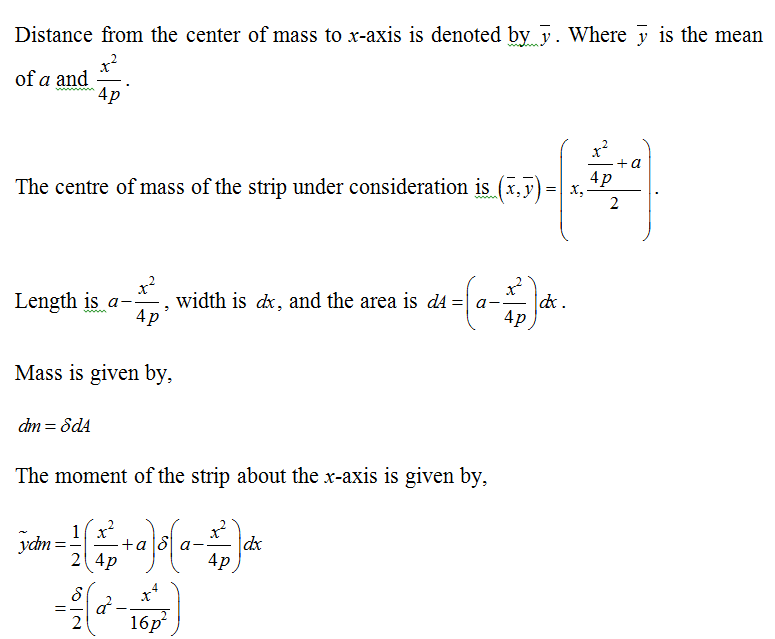# 42. Whatever the value of p > 0 in the equation y = x²/(4p), they-coordinate of the centroid of the parabolic segment shown hereis ỹ = (3/5)a.ayх||

Question
1 viewshelp_outlineImage Transcriptionclose42. Whatever the value of p > 0 in the equation y = x²/(4p), the y-coordinate of the centroid of the parabolic segment shown here is ỹ = (3/5)a. a y х || fullscreen
check_circle

Formula used:Calculation:Model the mass distribution using the vertical strips....

### Want to see the full answer?

See Solution

#### Want to see this answer and more?

Solutions are written by subject experts who are available 24/7. Questions are typically answered within 1 hour.*

See Solution
*Response times may vary by subject and question.
Tagged in